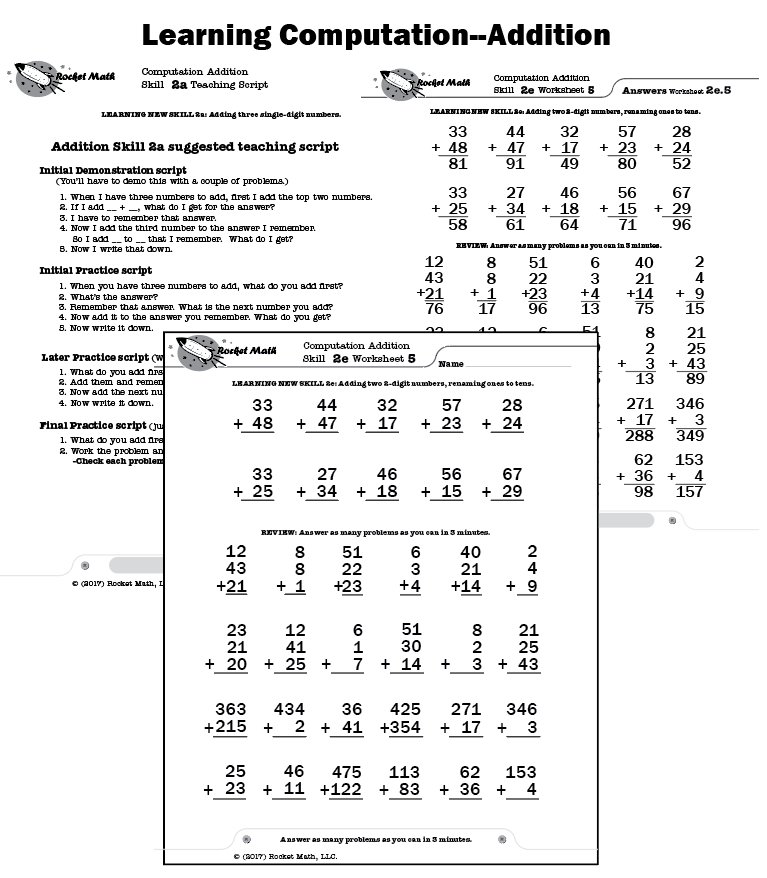## How to do (compute) multi-step addition problems

After becoming fluent with addition facts, the best way for students to retain the knowledge of those facts is by doing addition computation.  If students have not been taught addition computation, this program breaks it down into small, easy-to-learn steps that are numbered in a teaching sequence that leaves nothing to chance.

## Easily assess where to begin instruction

An assessment is provided to test each of the skills in the sequence below. They go in order. Test the student and begin teaching wherever the student begins to have difficulty.

## Skills taught in this Learning Track

Note that the number for each skill gives the grade level as well as indicating the teaching sequence.  Skill 2a is a 2nd grade skill and after skill 2f is learned the next in the sequence is skill 3a.  The sequence of skills is drawn from M. Stein, D. Kinder, J. Silbert, and D. W. Carnine, (2006) Designing Effective Mathematics Instruction: A Direct Instruction Approach (4th Edition) Pearson Education: Columbus, OH.

(1b) Adding 1-, or 2-digit numbers; no renaming

(2b-c) Adding 3-digit numbers; no renaming

(2c) Adding 3-digits to 1 or more digits; no renaming

(2d) Adding three 1- or 2-digit numbers; no renaming

(2e) Adding two 2-digit numbers, renaming 1s to 10s

(2f) Adding 3-digit numbers, renaming 1s to 10s

(3a) Adding a 1-digit number to a teen number, under 20

(3b) Adding two 2- or 3-digit numbers; renaming 10s to 100s

(3c) Adding 3-digit numbers; renaming twice

(3d) Adding three 2-digit numbers; renaming sums under 20

(3e) Adding four multi-digit numbers; renaming, sums under 20

(4a) Adding a 1-digit number to a teen number, over 20

(4b) Adding three 2-digit numbers, sums over 20

(4c) Adding four or five multi-digit numbers, sums over 20

## How to teach this Learning Track

For each skill there is a suggested Teaching Script giving the teacher/tutor/parent consistent (across all the skills we use the same explanation) language of instruction on how to do the skill.  The script helps walk the student through the computation process.  For the teacher, in addition to the script, there are answer keys for the five worksheets provided for each skill.

Each worksheet is composed of two parts.  The top has examples of the skill being learned that can be worked by following the script.  After working through those examples with the teacher the student is then asked to work some review problems of addition problems that are already known.  The student is asked to do as many as possible in 3 minutes—a kind of sprint.  If all is well the student should be able to do all the problems or nearly all of them, but finishing is not required.  Three minutes of review is sufficient for one day.

There are five worksheets for each skill.  Gradually as the student learns the skill the teacher/tutor/parent can provide progressively less help and the student should be able to do the problems without any guidance by the end of the five worksheets.  There are suggestions for how to give less help in the teaching scripts.